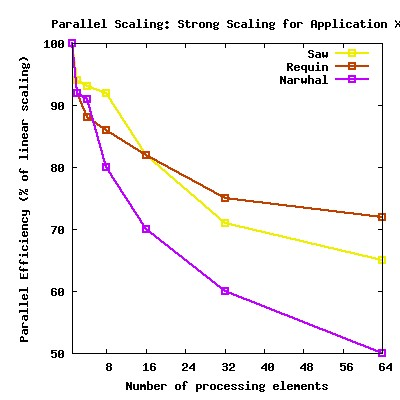## Parallel Scalability

A common task in HPC is measuring the scalability (also referred to as the scaling efficiency) of an application. This measurement indicates how efficient an application is when using increasing numbers of parallel processing elements (CPUs / cores / processes / threads / etc.).

There are two basic ways to measure the parallel performance of a given application, depending on whether or not one is cpu-bound or memory-bound. These are referred to as strong and weak scaling, respectively.

## Strong Scaling

In this case the problem size stays fixed but the number of processing elements are increased. This is used as justification for programs that take a long time to run (something that is cpu-bound). The goal in this case is to find a "sweet spot" that allows the computation to complete in a reasonable amount of time, yet does not waste too many cycles due to parallel overhead. In strong scaling, a program is considered to scale linearly if the speedup (in terms of work units completed per unit time) is equal to the number of processing elements used ( N ). In general, it is harder to achieve good strong-scaling at larger process counts since the communication overhead for many/most algorithms increases in proportion to the number of processes used.

### Calculating Strong Scaling Efficiency

If the amount of time to complete a work unit with 1 processing element is t1, and the amount of time to complete the same unit of work with N processing elements is tN, the strong scaling efficiency (as a percentage of linear) is given as:

t1 / ( N * tN ) * 100%

## Weak Scaling

In this case the problem size (workload) assigned to each processing element stays constant and additional elements are used to solve a larger total problem (one that wouldn't fit in RAM on a single node, for example). Therefore, this type of measurement is justification for programs that take a lot of memory or other system resources (something that is memory-bound). In the case of weak scaling, linear scaling is achieved if the run time stays constant while the workload is increased in direct proportion to the number of processors. Most programs running in this mode should scale well to larger core counts as they typically employ nearest-neighbour communication patterns where the communication overhead is relatively constant regardless of the number of processes used; exceptions include algorithms that employ heavy use of global communication patterns, eg. FFTs and transposes.

### Calculating Weak Scaling Efficiency

If the amount of time to complete a work unit with 1 processing element is t1, and the amount of time to complete N of the same work units with N processing elements is tN, the weak scaling efficiency (as a percentage of linear) is given as:

( t1 / tN ) * 100%

## Scaling Measurement Guidelines

Further to basic code performance and optimization concerns (ie. the single thread performance), one should consider the following when timing their application:

1. use wallclock time units or equivalent
• eg. timesteps completed per second, etc.
2. measure using multiple computer systems
• most importantly ones that have significantly different processor / network balances (ie. CPU speed vs. interconnect speed).
3. measure using job sizes that span:
• from 1 to the number of processing elements per node for threaded jobs
• from 1 to the total number of processes requested for MPI
• job size increments should be in power-of-2 or equivalent (cube powers for weak-scaling 3D simulations, for example)
• NOTE: it is inappropriate to refer to scaling numbers with more than 1 cpu as the baseline
• in scenarios where the memory requirements exceed what is available on a single node, one should provide scaling performance for smaller data-sets (lower resolution) so that scaling performance can be compared throughout the entire range from 1 to the number of processes they wish to use, or as close to this as possible, in addition to any results at the desired problem size
4. measure multiple independent runs per job size
• average results and remove outliers as appropriate
5. use a problem state or configuration that best matches your intended production runs
• scaling should be measured based on the overall performance of the application
• no simplified models or preferential configurations

Once you have timed your application you should convert the results to scaling efficiencies as explained above.

## Presenting Scaling Data: Examples

### Plot

Typically scaling efficiency is presented as a plot:### Table

Alternatively, if one is not familiar with plotting programs the raw measured durations and calculated scaling percentages can be presented in a tabular format, eg.:

```strong scaling efficiency for simulation X running for 1 hour
------------------------------------------------------------------
#cpu      requin timesteps   requin (%)  saw timesteps   saw (%)
1         159                100         223             100
2         300                94          410              96
4         589                93          800              94
8        1168                92         1596              93
16       2088                82         2800              85
32       3632                71         3600              69
64       6649                65         5600              55
```